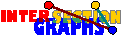# t-Intersection

The t-intersection graph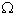t(H) of a hypergraph H=(A,Sx,xV) has V as vertex set, and two distinct vertices x,y are joined by an edge whenever |SxSy|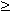t.

This concept has been introduced in [BHS77] and [HS76]. t-intersection makes only sense for discrete intersection models---the appropriate generalization for geometric models would be tolerance intersection.

The difficulty of the recognition problem seems higher than for ordinary intersection, since, as we shall see, in the duality approach we have a third step to tackle. Instead of the points of the hypergraph, now t-element point sets serve as detectors. For every such t-element subset M of A, we define M* as the set of those vV for which M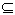Sv. Obviously each such M* induces a complete graph in the t-intersection graph G. Moreover, every edge of G is detected by some t-set of A. If we denote these complete graphs M* as the star graphs of G, then

the set of star graphs forms an edge cover by complete graphs of G.

But you should be aware that there is another dualization approach here, where still the single points are the detectors. Then detectors alone detect nothing, they work only in groups of t members.

For integers t1 and a hypergraph H=(A,(Sx/xV)), let its t-set hypergraph H[t] denote the hypergraph with those t-element subsets of A as vertices that occur in some hyperedge of H, and (Sx[t]/xV) as hyperedge family, where Sx[t] contains all t-element subsets B of A for which BSx. Now t-intersection graphs are just special intersection graphs:t(H) =(H[t]).

If H is t-linear (i.e. every two hyperedges have at most t points in common) and k-uniform, then H[t] is {k choose t}-uniform, and {t choose t}-linear. Therefore we get a generalization of a result in [LP92] and [JKL96] for t=t=k-1.t-intersection graphs of k-uniform t-linear hypergraphs are intersection graphs of certain {k choose t}-uniform, {t choose t}-linear hypergraphs.
For t2, not every {k choose t}-uniform, {t choose t}-linear hypergraph is the t-set hypergraph of some hypergraph, see the example to the right.
 S1={ 1, 2, 3, } S2={ 1, 4, 9, } S3={ 3, 8, 11, } S4={ 4, 5, 6, } S5={ 6, 7, 8, } S6={ 9, 10, 11, }

### k-Facet Graphs

Non-star cliques may be arbitrary large for intersection graphs of k-uniform hypergraphs and k3. Actually no such result is possible for t-intersection graphs of simple k-uniform hypergraphs and t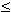k-2. Only for t = k-1 it works:[LP92]: Let k2, and let the k-sets X1, X2,...,Xr have pairwise k-1 elements in common. Then either the total intersection of these r sets contains k-1 elements, or |Xi|=k+1.

Therefore, every clique of size at least k+2 in the (k-1)-intersection graph of some simple (!) k-uniform hypergraph is a star graph. This should justify to give this concept a try. (k-1)-intersection graphs of simple k-uniform hypergraphs are abbreviated as k-facet graphs. Recall that every k-facet graph is the intersection graph of some linear k-uniform hypergraph.

Star graphs can be revealed for k=2 but not for k3.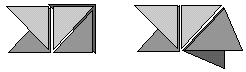However, it works almost. Let Sx and Sy be hyperedges of the k-uniform hypergraph H with |SxSy| =k-1. In the k-facet graph, the edge xy can be extended in only one way to a star graph clique, namely by adding all vertices z whose set Sz includes SxSy. Also, since |Sx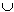Sy| =k+1, by the above lemma there is at most one way to extend xy to some non star graph clique, namely by adding all z whose Sz is contained in SxSy. Therefore, two star graphs in a k-facet graph have at most one common vertex, and two non star graph cliques have also at most one common vertex. Unfortunately, a star graph and a non star graph clique may have 0,1, or 2 common vertices, so there remains some ambiguity (which, however, has small probability).

There is at least a related problem where the star graph identification step works. The k-line graph of a graph is the (k-1)-intersection graph of the set of all Kks. In other words, k-line graphs are just the k-facet graphs of k-uniform k-conformal hypergraphs. Star cliques and non star graph cliques have 0 or 2 common vertices, thus again we have only two possibilities for the set of star graphs (since CM(G) is again connected).

The general problem is really hard:[JKL?]: For every k3 it is NP-complete to decide whether a triangle-free graphs is a k-facet graph.

Whether this difficulty is due to the difficulties of revealing star graphs or to the difficulty of recognizing t-set hypergraphs, I do not know.What is the complexity of the problem to recognize t-set hypergraphs of (t+1)-uniform hypergraphs, for t2? What is the complexity of recognizing k-line graphs?

Back to the start page for intersection graphs.

Erich Prisner